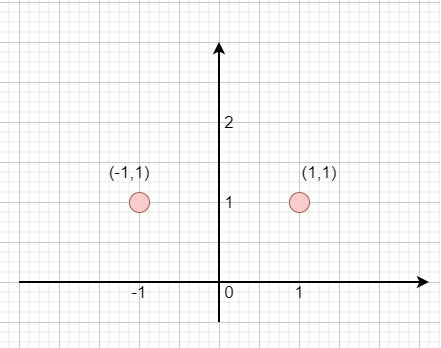# Line Reflection in C++

Suppose we have n points on a 2D plane, we have to check whether there is any line parallel to y-axis that reflect the given points symmetrically, in other words, check whether there exists a line that after reflecting all points over the given line the set of the original points is the same that the reflected ones.

So, if the input is like points = [[1,1],[-1,1]]then the output will be true

To solve this, we will follow these steps −

• Define one set ok

• n := size of points

• minVal := inf

• maxVal := -inf

• for initialize i := 0, when i < n, update (increase i by 1), do −

• minVal := minimum of minVal and points[i, 0]

• maxVal := maximum of maxVal and points[i, 0]

• insert points[i] into ok

• mid := maxVal + minVal

• for initialize i := 0, when i < n, update (increase i by 1), do −

• x := points[i, 0]

• y := points[i, 1]

• x := mid - x

• if { x, y } is not in ok, then −

• return false

• return true

## Example

Let us see the following implementation to get better understanding −

Live Demo

#include <bits/stdc++.h>
using namespace std;
class Solution {
public:
bool isReflected(vector<vector<int<>& points) {
set<vector<int< > ok;
int n = points.size();
int minVal = INT_MAX;
int maxVal = INT_MIN;
for (int i = 0; i < n; i++) {
minVal = min(minVal, points[i]);
maxVal = max(maxVal, points[i]);
ok.insert(points[i]);
}
int mid = maxVal + minVal;
for (int i = 0; i < n; i++) {
int x = points[i];
int y = points[i];
x = mid - x;
if (!ok.count({ x, y }))
return false;
}
return true;
}
};
main(){
Solution ob;
vector<vector<int<> v = {{1,1},{-1,1}};
cout << (ob.isReflected(v));
}

## Input

{{1,1},{-1,1}}

## Output

1